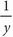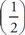# SAT Math Multiple Choice Question 173: Answer and Explanation

### Test Information

Question: 173

8. Environmentalists have been monitoring the area of a glacier in Canada. The glacier is slowly shrinking. The glacier originally occupied 15,000 square miles, but after two years of monitoring the glacier, the scientists document that the area of the glacier is now 14,910 square miles. If y is the number of years since monitoring began, which equation best describes the glacier's area, G(y), as a function of time?

• A. G(y) = 15,000• B. G(y) = 15,000 (0.003)y
• C. G(y) = 15,000(0.997)y
• D. G(y) = 0.997y

C Plug 2 in for y in the answers to see which function most closely equals the area of 14,910. Choice (A) becomes 15,000= 7,500. This does not match the target number, so eliminate (A). Choice (B) becomes 15,000(0.003)2 = 0.135. Eliminate (B). Choice (C) becomes 15,000(0.997)2 ≈ 14,910. The correct answer is (C).